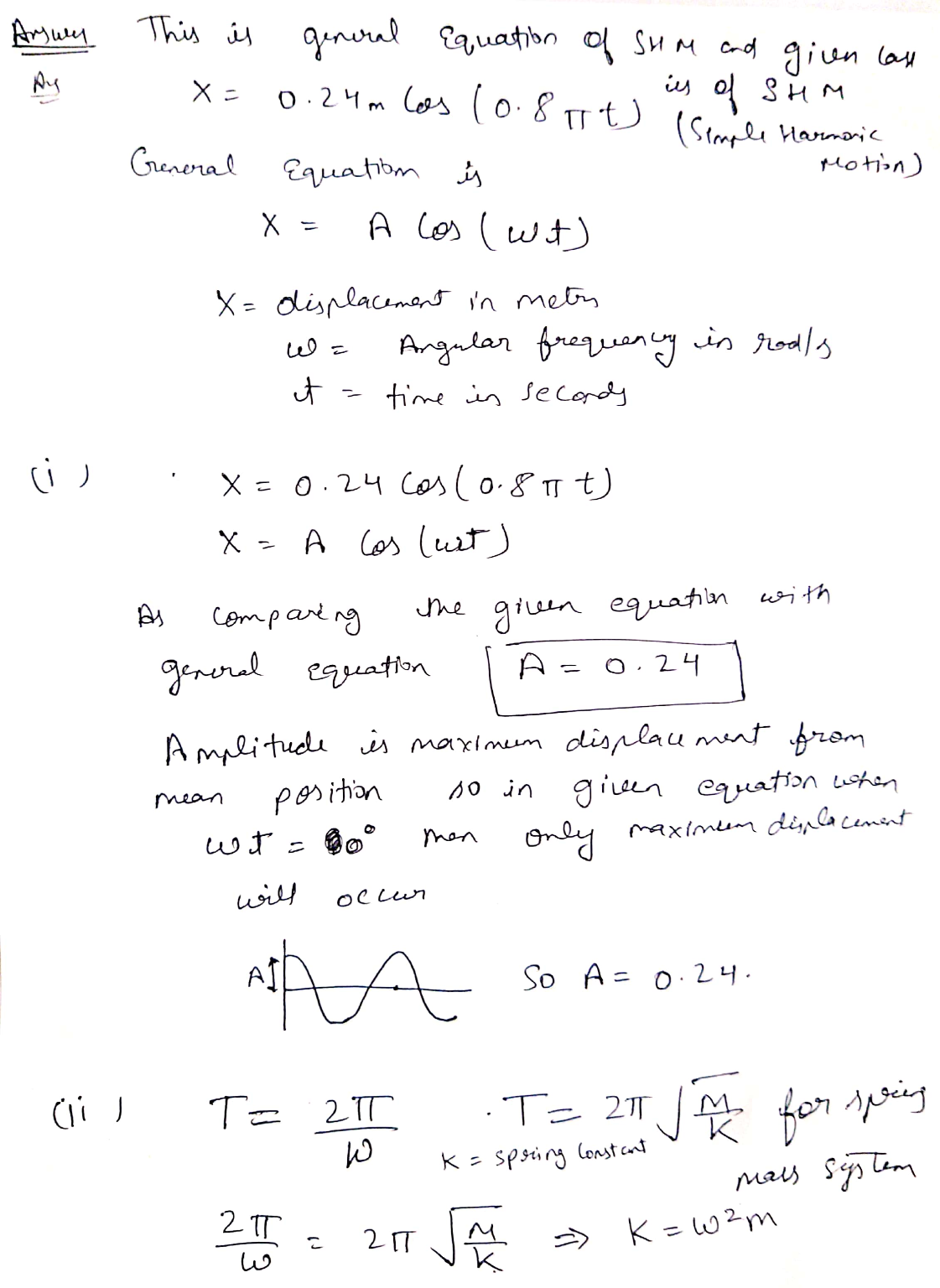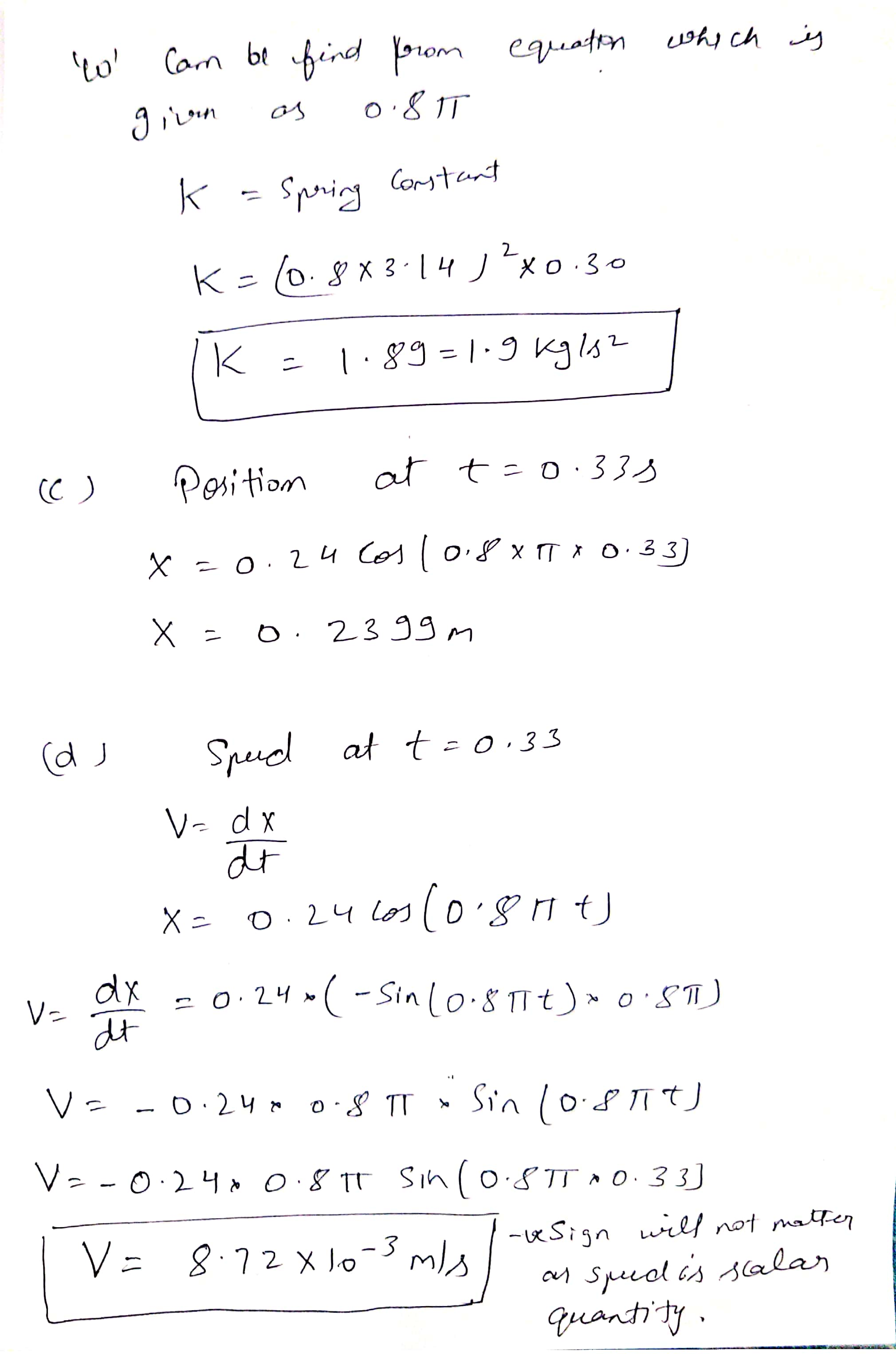In: Physics

# The position of a 0.30-kg object attached to a spring is described by x = (0.24...

The position of a 0.30-kg object attached to a spring is described by x = (0.24 m) cos(0.8πt). (Assume t is in seconds.) (a) Find the amplitude of the motion. 0.24 Correct: Your answer is correct. m (b) Find the spring constant. 1.9 Correct: Your answer is correct. N/m (c) Find the position of the object at t = 0.33 s. 0.16 Correct: Your answer is correct. m (d) Find the object's speed at t = 0.33 s. Incorrect: Your answer is incorrect.

## Solutions

##### Expert Solution## Related Solutions

##### A mass of 0.30 kg is attached to a spring and set into oscillation on a...
A mass of 0.30 kg is attached to a spring and set into oscillation on a horizontal frictionless surface. The simple harmonic motion of the mass is described by x(t) = (0.48 m)cos[(8 rad/s)t]. Determine the following. (a) amplitude of oscillation for the oscillating mass. Answer in m (b) force constant for the spring. Answer in N/m (c) position of the mass after it has been oscillating for one half a period. Answer in m (d) position of the mass...
##### In a horizontal spring-mass configuration, the position of the 0.44 kg mass is described by [x...
In a horizontal spring-mass configuration, the position of the 0.44 kg mass is described by [x = (0.49 m) sin(2.39t)] a) What is in meters the amplitude of these oscillations? b) What is in m/s the maximum speed of the mass under these conditions? c) What is in Joules the spring potential energy stored in this system when the spring is stretched at a maximum? d) What is in Joules the kinetic energy of the mass when the spring is...
##### An object with mass 3.5 kg is attached to a spring with spring stiffness constant k...
An object with mass 3.5 kg is attached to a spring with spring stiffness constant k = 270 N/m and is executing simple harmonic motion. When the object is 0.020 m from its equilibrium position, it is moving with a speed of 0.55 m/s.(a) Calculate the amplitude of the motion._____ m(b) Calculate the maximum velocity attained by the object. [Hint: Use conservation of energy.]______ m/s
##### an 10 kg object is hung from a spring attached to a fixed support. The spring...
an 10 kg object is hung from a spring attached to a fixed support. The spring constant of the spring is k=40 Nm**(-1) (I mean to the power of -1) Suppose an external downward force of magnitude f(T) = 20 e **(-2t) N is applied to the object, and damping due to air resistence occurs with damping constant beta = 40 N s m **(-1). Let y(t) denote the distance in meters of the object below its equilibrium position at...
##### An object attached to a spring vibrates with simple harmonic motion as described by the figure...
An object attached to a spring vibrates with simple harmonic motion as described by the figure below. (a) For this motion, find the amplitude.   (b) For this motion, find the period. (c) For this motion, find the angular frequency.  (d) For this motion, find the maximum speed  (e) For this motion, find the maximum acceleration.  (f) For this motion, find an equation for its position x in terms of a sine function.
##### A 6.0 kg object attached to a horizontal spring oscillates with an amplitude A = 10...
A 6.0 kg object attached to a horizontal spring oscillates with an amplitude A = 10 cm and a frequency f = 2.2 Hz. (a) What is the force constant of the spring? _____N/m (b) What is the period of the motion? _____s (c) What is the maximum speed of the object? _____m/s (d) What is the maximum acceleration of the object? _____m/s2
##### A 2.20-kg object is attached to a spring and placed on frictionless, horizontal surface. A horizontal...
A 2.20-kg object is attached to a spring and placed on frictionless, horizontal surface. A horizontal force of 18.0 N is required to hold the object at rest when it is pulled 0.200 m from its equilibrium position (the origin of the x axis). The object is now released from rest from this stretched position, and it subsequently undergoes simple harmonic oscillations. (a) Find the force constant of the spring. N/m (b) Find the frequency of the oscillations. Hz (c)...
##### A 3kg object is attached to spring and will stretch the spring 392mm by itself. There...
A 3kg object is attached to spring and will stretch the spring 392mm by itself. There is no damping in the system and a forcing function of F(t)=10 coswt, w=(k/w)-2 is attached to the object. The spring is deflected y distance father than equilibrium position due to the F(t). k is the spring modulus and M is the mass of the object. a) Derive the governing equation for the spring-object mass system decribed in the problem.Assume Mg=hk b) if the...
##### A block attached to a spring is displaced from equilibrium to the position x=+1.3m and released....
A block attached to a spring is displaced from equilibrium to the position x=+1.3m and released. The period is 2.6s. At what positions and times during the first complete cycle do the following conditions occur. a) |v|=0.5vmax|, Where vmax is the maximum speed? First position:  m . . . First time:  s. Second position:  m . . . Second time:  s. Third position:  m . . . Third time:  s. Fourth position:  m . . . Fourth time:  s. b) |a|=0.5amax. Where amax is the maximum magnitude of...
##### A mass of 0.30 kg on the end of a spring oscillates with a period of...
A mass of 0.30 kg on the end of a spring oscillates with a period of 0.45 s and an amplitude of 0.15 m . A) Find the velocity when it passes the equilibrium point. B) Find the total energy of the system. C) Find the spring constant. D) Find the maximum acceleration of the mass.